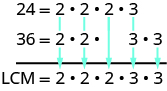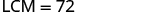# 1.1 Introduction to whole numbers  (Page 6/18)

 Page 6 / 18

Find the Least Common Multiple (LCM) of 24 and 36 using the prime factors method.

## Solution

 Find the primes of 24 and 36. Match primes vertically when possible. Bring down all columns.Multiply the factors.The LCM of 24 and 36 is 72.

Find the LCM using the prime factors method: 21 and 28.

84

Find the LCM using the prime factors method: 24 and 32.

96

## Key concepts

• Place Value as in [link] .
• Name a Whole Number in Words
1. Start at the left and name the number in each period, followed by the period name.
2. Put commas in the number to separate the periods.
3. Do not name the ones period.
• Write a Whole Number Using Digits
1. Identify the words that indicate periods. (Remember the ones period is never named.)
2. Draw 3 blanks to indicate the number of places needed in each period. Separate the periods by commas.
3. Name the number in each period and place the digits in the correct place value position.
• Round Whole Numbers
1. Locate the given place value and mark it with an arrow. All digits to the left of the arrow do not change.
2. Underline the digit to the right of the given place value.
3. Is this digit greater than or equal to 5?
• Yes—add 1 to the digit in the given place value.
• No—do not change the digit in the given place value.
4. Replace all digits to the right of the given place value with zeros.
• Divisibility Tests: A number is divisible by:
• 2 if the last digit is 0, 2, 4, 6, or 8.
• 3 if the sum of the digits is divisible by 3.
• 5 if the last digit is 5 or 0.
• 6 if it is divisible by both 2 and 3.
• 10 if it ends with 0.
• Find the Prime Factorization of a Composite Number
1. Find two factors whose product is the given number, and use these numbers to create two branches.
2. If a factor is prime, that branch is complete. Circle the prime, like a bud on the tree.
3. If a factor is not prime, write it as the product of two factors and continue the process.
4. Write the composite number as the product of all the circled primes.
• Find the Least Common Multiple by Listing Multiples
1. List several multiples of each number.
2. Look for the smallest number that appears on both lists.
3. This number is the LCM.
• Find the Least Common Multiple Using the Prime Factors Method
1. Write each number as a product of primes.
2. List the primes of each number. Match primes vertically when possible.
3. Bring down the columns.
4. Multiply the factors.

## Practice makes perfect

Use Place Value with Whole Numbers

In the following exercises, find the place value of each digit in the given numbers.

51,493
1
4
9
5
3

thousands hundreds tens ten thousands ones

87,210
2
8
0
7
1

164,285
5
6
1
8
2

ones ten thousands hundred thousands tens hundreds

395,076
5
3
7
0
9

93,285,170
9
8
7
5
3

ten millions ten thousands tens thousands millions

36,084,215
8
6
5
4
3

7,284,915,860,132
7
4
5
3
0

trillions billions millions tens thousands

2,850,361,159,433
9
8
6
4
2

In the following exercises, name each number using words.

1,078

one thousand, seventy-eight

hello, I have algebra phobia. Subtracting negative numbers always seem to get me confused.
what do you need help in?
Felix
Heather
look at the numbers if they have different signs, it's like subtracting....but you keep the sign of the largest number...
Felix
for example.... -19 + 7.... different signs...subtract.... 12 keep the sign of the "largest" number 19 is bigger than 7.... 19 has the negative sign... Therefore, -12 is your answer...
Felix
—12
Thanks Felix.l also get confused with signs.
Esther
Thank you for this
Shatey
Bruce drives his car for his job. The equation R=0.575m+42 models the relation between the amount in dollars, R, that he is reimbursed and the number of miles, m, he drives in one day. Find the amount Bruce is reimbursed on a day when he drives 220 miles
168.50=R
Heather
john is 5years older than wanjiru.the sum of their years is27years.what is the age of each
46
mustee
j 17 w 11
Joseph
john is 16. wanjiru is 11.
Felix
27-5=22 22÷2=11 11+5=16
Joyce
I don't see where the answers are.
Ed
Cindy and Richard leave their dorm in Charleston at the same time. Cindy rides her bicycle north at a speed of 18 miles per hour. Richard rides his bicycle south at a speed of 14 miles per hour. How long will it take them to be 96 miles apart?
3
Christopher
18t+14t=96 32t=96 32/96 3
Christopher
show that a^n-b^2n is divisible by a-b
What does 3 times your weight right now
Use algebra to combine 39×5 and the half sum of travel of 59+30
Cherokee
What is the segment of 13? Explain
Cherokee
my weight is 49. So 3 times is 147
Cherokee
kg to lbs you goin to convert 2.2 or one if the same unit your going to time your body weight by 3. example if my body weight is 210lb. what would be my weight if I was 3 times as much in kg. that's you do 210 x3 = 630lb. then 630 x 2.2= .... hope this helps
tyler
How to convert grams to pounds?
paul
What is the lcm of 340
Yes
Cherokee
How many numbers each equal to y must be taken to make 15xy
15x
Martin
15x
Asamoah
15x
Hugo
1y
Tom
1y x 15y
Tom
find the equation whose roots are 1 and 2
(x - 2)(x -1)=0 so equation is x^2-x+2=0
Ranu
I believe it's x^2-3x+2
NerdNamedGerg
because the X's multiply by the -2 and the -1 and than combine like terms
NerdNamedGerg
find the equation whose roots are -1 and 4
Ans = ×^2-3×+2
Gee
find the equation whose roots are -2 and -1
(×+1)(×-4) = x^2-3×-4
Gee
yeah
Asamoah
there's a chatting option in the app wow
Nana
That's cool cool
Nana
Nice to meet you all
Nana
you too.
Joan
😃
Nana
Hey you all there are several Free Apps that can really help you to better solve type Equations.
Debra
Debra, which apps specifically. ..?
Nana
am having a course in elementary algebra ,any recommendations ?
samuel
Samuel Addai, me too at ucc elementary algebra as part of my core subjects in science
Nana
me too as part of my core subjects in R M E
Ken
at ABETIFI COLLEGE OF EDUCATION
Ken
ok great. Good to know.
Joan
5x + 1/3= 2x + 1/2
sanam
Plz solve this
sanam
5x - 3x = 1/2 - 1/3 2x = 1/6 x = 1/12
Ranu
Thks ranu
sanam
Erica
the previous equation should be 3x = 1/6 x=1/18
Sriram
for the new one 10x + 2x = 38 - 14
Sriram
12x = 24 x=2
Sriram
10x + 14 = -2x +38 10x + 2x = 38 - 14 12x = 24 divide both sides by the coefficient of x, which is 12 therefore × = 2
vida
a trader gains 20 rupees loses 42 rupees and then gains ten rupees Express algebraically the result of his transactions
a trader gains 20 rupees loses 42 rupees and then gains 10 rupees Express algebraically the result of his three transactions
vinaya
a trader gains 20 rupees loses 42 rupees and then gains 10 rupees Express algebraically the result of his three transactions
vinaya
a trader gains 20 rupees loses 42 rupees and then gains 10 rupees Express algebraically the result of his three transactions
vinaya
Kim is making eight gallons of punch from fruit juice and soda. The fruit juice costs \$6.04 per gallon and the soda costs \$4.28 per gallon. How much fruit juice and how much soda should she use so that the punch costs \$5.71 per gallon?
(a+b)(p+q+r)(b+c)(p+q+r)(c+a) (p+q+r)
really
Asamoah
4x-7y=8 2x-7y=1 what is the answer?
x=7/2 & y=6/7
Pbp
x=7/2 & y=6/7 use Elimination
Debra
true
bismark
factoriz e
usman
4x-7y=8 X=7/4y+2 and 2x-7y=1 x=7/2y+1/2
Peggie
Frank
thanks
Ramil
x=7/2 y=6/7
Asamoah
Thanks , all of you are correct.
JosephByBy RhodesBy Sebastian Sieczko...